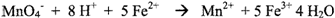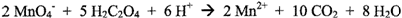Redox Titration Lab

Purpose

To determine the percent by mass of iron in the given sample by using the titration method

Introduction

Reduction/oxidation (redox) processes occur when electrons are transferred from a donor species (the reducing agent) to another acceptor species (the oxidizing agent). The loss of electrons is oxidation; the gain of electrons is reduction. Transfer of electrons can be illustrated by the following simple example:

Zn(s) + Cu2+ (aq) Zn2+ (aq) + Cu(s)

The plating of solid copper from solution on a zinc surface is actually two chemical processes, complementary to each other and occurring simultaneously:

Zn(s) → Zn2+ (aq) + 2e- (oxidation)

Cu2+(aq) + 2e- → Cu(s) (reduction)

The zinc metal loses electrons (is oxidized) to become aqueous zinc ion, while the copper ions gain electrons (are reduced) to become uncharged copper metal atoms. Equations that describe only the oxidation or reduction process are called half-reactions. Half- reactions are a useful means of balancing redox equations.

In this experiment you will use a redox reaction between iron (II) ions and permanganate (MnO4-) ions to determine the percent iron by mass in an unknown sample. The reaction can be partially described by the BALANCED equation shown below:The H+ indicates that this reaction occurs under acidic conditions. In this reaction, electrons are transferred from the iron (II) ion to the permanganate ion, liberating manganese (II) ion and oxidizing the iron (II) to iron (III). The permanganate ion is deeply colored (purple); the manganese (II) ion is colorless. As permanganate is added, more iron (II) ion is consumed, generating more manganese (II) ion and causing the color of the permanganate to disappear. When all the iron (II) is oxidized to iron (III), any additional permanganate will impart a permanent color to the solution because it will have nothing to react with. This is the end-point of the titration.

The potassium permanganate is standardized against sodium oxalate in acid solution:Potassium permanganate acts as its own indicator both in the standardization and in the iron determination,

1.      How many moles of Fe ion can be oxidized by 0.012 moles MnO4- ion in the reaction? Show work.

2.      A solid sample containing some Fe2+ ion weighs 1.923 g. It requires 36.44 mL of 0.0244 M KMnO4 to titrate the Fe2+ in the dissolved sample to a pink end point. Calculate the grams of iron in the sample and the percentage of iron in the sample. Show work with units.

3.      What is the percentage of iron in iron (II) ammonium sulfate hexahydrate? Show work.
(Hint - this is obtained from the formula of the compound.)

Procedure

A.    Preparation of the potassium permanganate solution

Prepare 250 mL of 0.1 M KMn04 solution in a 250 mL standard flask.

B.     Preparation of the sodium oxalate solution

Prepare 100 mL of 0.25 M Na2C204 solution in a 100 mL standard flask.

NOTE:

1. The potassium permanganate solution must be used when freshly made to obtain accurate results. Therefore, this entire experiment must be completed on the same day.

2. Add the permanganate directly into the oxalate solution (not down the walls ofthe beaker). Promptly wash down any KMn04 that spatters on the walls of the beaker into the bulk of the liquid using a wash bottle.

3. Finely divided MnO2 will form if the KMnO4 is added too rapidly and will cause the solution to acquire a faint brown discoloring. This is not a serious problem of sufficient oxylate remains to reduce the MnO2 to Mn2+; simply discontinue the titration until the brown color disappears.

4. The surface of the permanganate solution rather that the bottom of the meniscus can be used to measure titrant volumes.

5. Partial decomposition of the permanganate to MnO2 may occur if it remains in the buret for a long time. Clean the buret with a dilute sodium bisulfite solution.

C.    Standardization of potassium permanganate solution with sodium oxalate solution

Pipet out 20 mL of 0.25 M sodium oxalate solution into an Erlenmeyer flask. Add 15 mL of 3 M H2S04 to the flask and heat to about 80 - 90°C. Titrate the hot solution with potassium permanganate while stirring with a magnetic stirrer. The pink color imparted by one addition should be permitted to disappear before further titrant is added. The temperature should not drop below 60°C. The end point is marked by the appearance of a faint pink color that persists at least 30 seconds.

D.    Analysis of the give iron compound by titration using the standardized potassium permanganate solution

You will get an unknown iron sample from your instructor. Weigh out 0.2 g into a 250 mL Erlenmeyer flask. Dissolve the sample by adding about 15 mL of deionized water and then add 15 mL of 3M sulfuric acid. Titrate the unknown samples as you did in part C. (You need not heat the solution). Stop titrating when a permanent pale pink color appears. Record initial and final buret readings for each sample.

1.     Calculate the molarity of the permanganate solution using the standard sodium oxalate solutions molarity and the titer value.

2.     Moles of permanganate ion used in part D =

3.     Moles of iron ion present in the sample =

4.     Mass of iron ion present in the sample =

5.     Fe present in the sample =

Post lab Questions:

1.      Discuss three sources of error and improvements to the errors that you discuss.

2.      How would these actions affect your calculated value of the moles of the iron?

a.     When you finished a titration and had recorded your data, you noticed small drops of the solution still clinging to spots on the inside walls of the buret near the top of the buret.

b.     You added the KMnO4 rapidly at the beginning and the solution had a slightly muddy brown color that would not go away. Since it was not very dark, you continued the titration to the faint pink end point.

c.     Potassium permanganate will slowly decompose in strong sunlight to MnO2 and is usually stored in dark glass bottles. You standardized your KMnO4 one day, and stored it in a clear bottle on the window sill. Several days later you used this solution to determine the iron.

d.    When you were adding drops of KMnO4 solution to the Erlenmeyer flask, a drop of the solution fell in after you reached the end point.

e.     If you did not add the H2SO4 solution to one of the wells but added H2O. During the titration the solution turned muddy brown.

3.      What chemistry themes apply to this lab?